# Proof that Z^2 = -1 cannot be solved on real spaces with an odd dimension. (General theory part 1.)

Finally after all those years something of a more general approach to multiplication in higher dimensions? Yes but at the same time I remark you should not learn or study higher dimensional numbers that way. You better pick a particular space like 3D complex numbers and find a lot out about them and then move on to say 4D or 5D complex numbers and repeat that process.
Problem with a more general approach is that those spaces are just too different from each other so it is hard to find some stuff all of those spaces have. It is like making theory for the complex plane and the split complex numbers at the same time: It is not a good idea because they behave very differently.
The math in this post is utterly simple, basically I use only that the square of a real number, this time a determinant, cannot be negative. The most complicated thing I use of the rule that says the determinant of a square is the square of the determinant like in det(Z^2) = det(Z)^2.

This post is only 3.5 pictures long so I added some extra stuff like the number tau for the 4D complex numbers and my old proof from 2015 that on the space of 3D complex numbers you can’t solve X^2 = -1.

I hope it’s all a bit readable so here we go: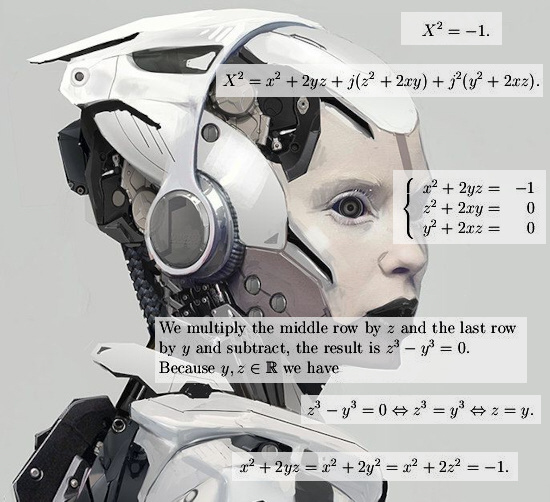Oops, this is the circular multiplication… Well replace j^3 = 1 by j^3 = -1 and do it yourself if you want to.

So all in all my goal was to use the impossibility of x^2 being negative on the real line to the more general setting of n-dimensional numbers. As such the math in this post is not very deep, it is as shallow as possible. Ok ok may be that 4D tau is some stuff that makes math professors see water burning because they only have the complex plane.
Let me end this post with thanking you to make it till the end, you have endured weird looking robots without getting mentally ill! Congratulations!
At the end a link to that old file from 2015:

# An important calculation of the 7D number tau (circular version).

I really took the time to compose this post; basically it is not extremely difficult to understand. Everybody who once has done matrix diagonalization and is still familiar with the diverse concepts and ideas around that can understand what we are doing here.

It is the fact that it is seven dimensional that makes it hard to write down the calculations in a transparent manner. I think I have succeeded in that detail of transparency because at the end we have to multiply three of those large seven by seven matrices with each other and mostly that is asking for loosing oversight.

Luckily one of those matrices is a diagonal matrix and with a tiny trick we can avoid the bulk of the matrix calculations by calculating the conjugate of the number tau.

Just like in the complex plane where the conjugate of the number i equals -i, for tau goes the same.

Basically the numbers tau are always the logarithm of the first imaginary component. But check if the determinant is one because you can use the tau to craft an exponential curve that will go through all basis vectors with determinant one.

This post is 10 pictures long (size 550 x 775), in the beginning I use an applet for the numerical calculation of the matrix representation of the first imaginary unit in 7D space, here is the link:

Matrix logarithm calculator
http://calculator.vhex.net/calculator/linear-algebra/matrix-logarithm

Two years back in 2015 after I found the five dimensional numbers tau every now and then I typed in a higher dimensional imaginary unit and after that only staring at the screen of the computer: How to find those numbers as the log applet says…

The method as shown here can be applied in all dimensions and you now have a standard way of crafting exponential curves in all spaces you want. This method together with the modified Dirichlet kernels that provide always a parametrization of the exponential curve form a complete description.
Ok ok those modified Dirichlet kernels always have period pi while this way to calculation the log of the first imaginary unit is always related to the dimension (recall that the 7D first imaginary unit l has the property l^7 while for the complex multiplication in 7D space we have l^7 = -1), but it is very easy to fix the Dirichlet kernels to the proper period in the time domain you want.

The most difficult part of this post is in understanding the subtle choice for the eigenvalues of tau = log l, or better; choosing the eigenvalues of the matrix representation involved. That makes or breaks this method, if done wrong you end up with a giant pile of nonsense…

Have fun reading it and if this is your first time you encounter those matrices with all these roots of unity in them, take your time and once more: take your time.
If you have never seen a matrix like that it is very hard to understand this post in only one reading…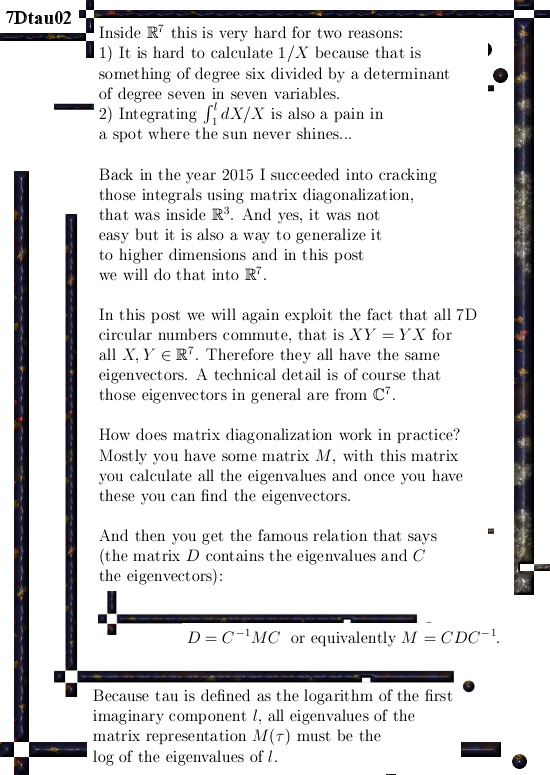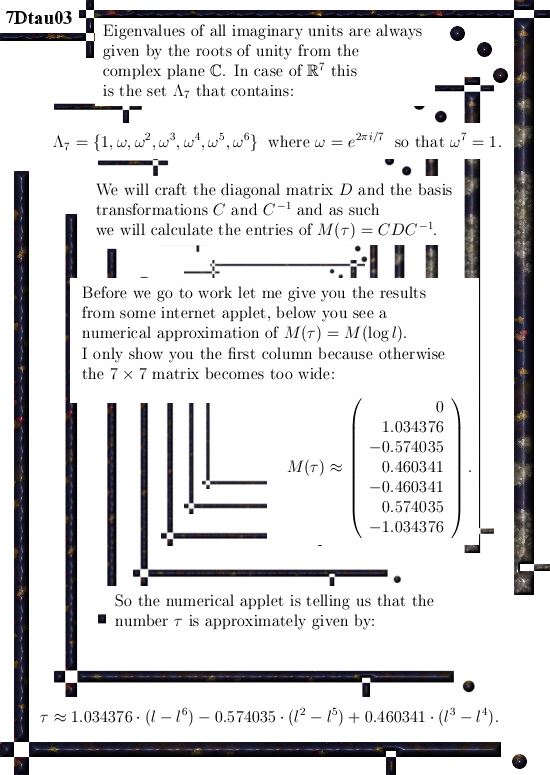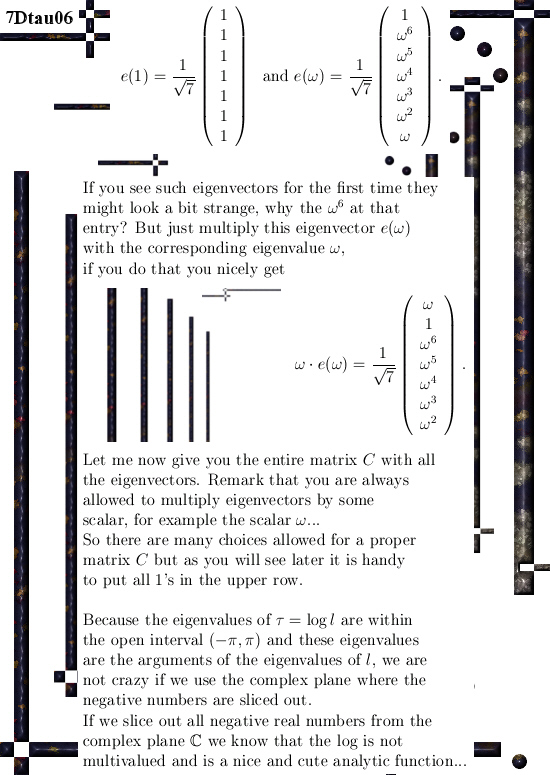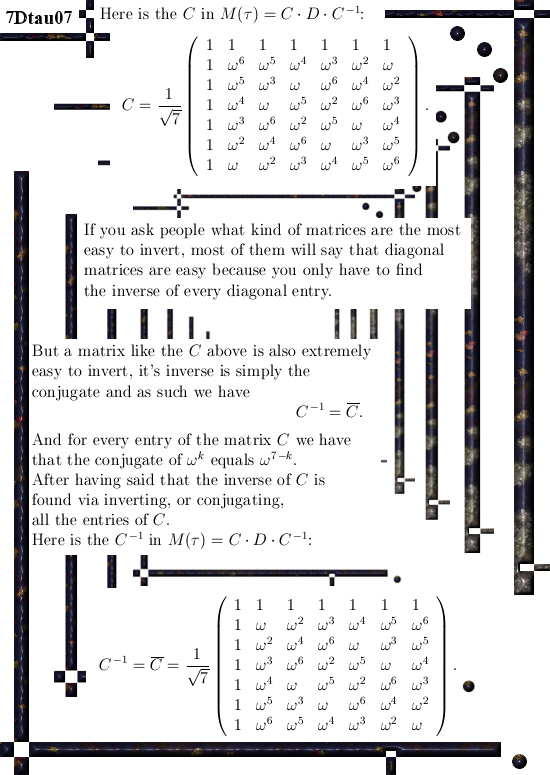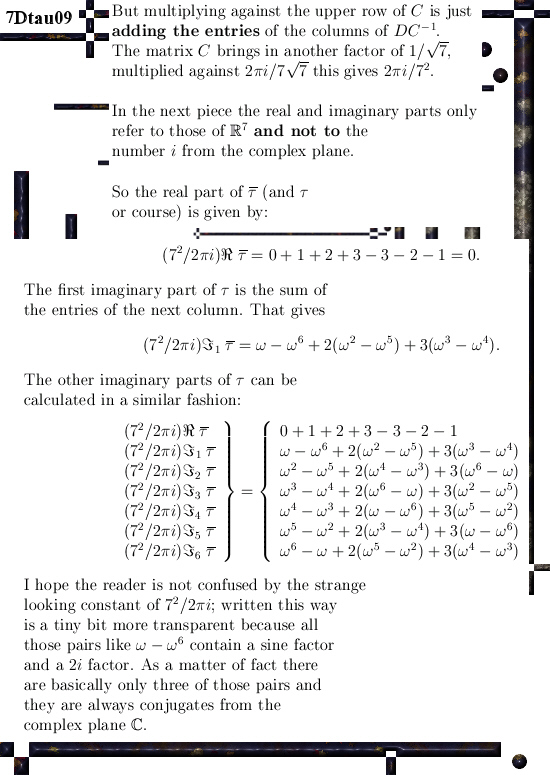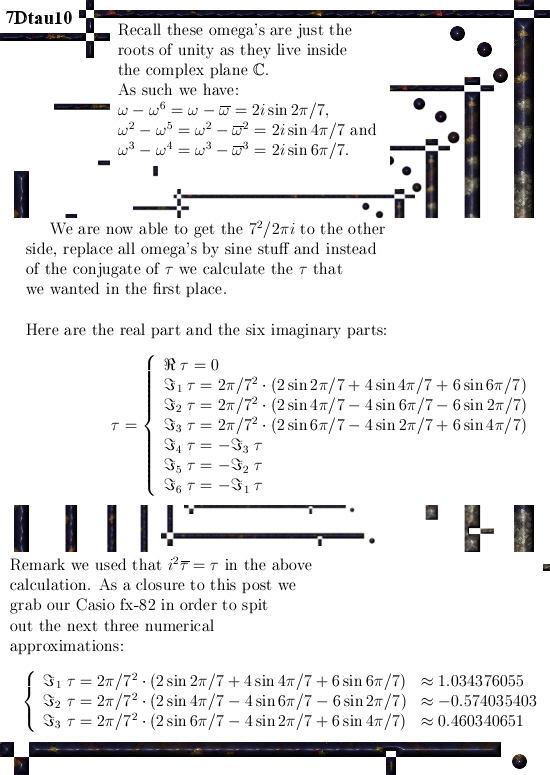I am glad all that staring to those numerical values is over and we have the onset of analytical understanding of how they are in terms of the angle 2 pi over 7.
The result is far from trivial; with the three or five dimensional case you can use other ways but the higher the dimension becomes the harder it gets.

This method that strongly relies on finding the correct diagonal matrix only becomes more difficult because the size of the matrices grows. So only the execution of the calculation becomes more cumbersome, the basic idea stays the same.

__________

I have no idea what the next post is going to be, may be a bit of magnetism because a few days back I got some good idea in explaining the behaviour of solar plasma included all those giant rings that shoot up and land in another spot of the sun.

And we also have those results from the Juno mission to Jupiter where the electrons also come from Jupiter itself without the guidance of electrical fields. But in the preprint archive I still cannot find only one work about it, that might be logical because often people do not write about stuff they don’t understand…

Ok, that was it. I hope you liked it & see ya around.

# Intro to the calculation of the seven dimensional number tau (circular version).

All details will be in the next post but I succeeded into using matrix diagonalization in order to find this seven dimensional number tau.
For people who do not understand what a number tau is, this is always the logarithm of an imaginary unit. Think for example at the complex plane and her imaginary unit i. The number tau for the complex plane is log i = i pi/2.

The problem with finding numbers tau becomes increasingly difficult as the number of dimensions rise. I remember back in the year 2015 just staring at all those matrices popping up using internet applets like the next one:

Matrix logarithm calculator (it uses the de Pade approximation)
http://calculator.vhex.net/calculator/linear-algebra/matrix-logarithm

Yet back in the year 2015 I was riding on my noble iron horse (a cheap bicycle) through the swamps surrounding the village of Haren and suddenly I had a good idea. Coming home I tried the idea of matrix diagonalization out in 3 dimensions and it worked.

I even wrote a post about it on 23 Nov 2015:

Integral calculus done with matrix diagonalization.

Now I think that most readers who visit this website are familiar with the concept of finding a diagonal matrix D containing all eigenvalues of a given matrix M. Once you have the eigenvalues you can calculate the eigenvectors and as such craft your matrix C containing all eigenvectors.
You can write the stuff as next: D = C^-1 M C.

Suppose you don’t know what M is but I give you the matrices D, C and the inverse of C. Can you find the matrix M?

Yes that is a beerwalk, all you have to do is calculate M = C D C^-1 and you are good to go.

But with the logarithm comes a whole lot of subtle things for making the right choice for the eigenvalues that you place inside the diagonal matrix D. It turns out you only get the desired result if you use arguments in the complex plane between minus and plus pi.
This is caused by the fact that you always need to make a cut in the complex plane if you want to work with the complex logarithm; but it is a bit surprising that only the cut where you leave out all real negative numbers (and zero of course) makes the calculation go perfect and in all other cases it ends in utter and total disaster.

In the next three pictures I show you some screen shots with numerical values of matrix representations and the logarithm of those matrix representations.

The goal is to find mathematical expressions for the observed numerical values that are calculated via the above mentioned de Pade approximation. We don’t want only numerical approximations but also catch the stuff in a mathematical formulation.

At the end of the third picture you see the end result.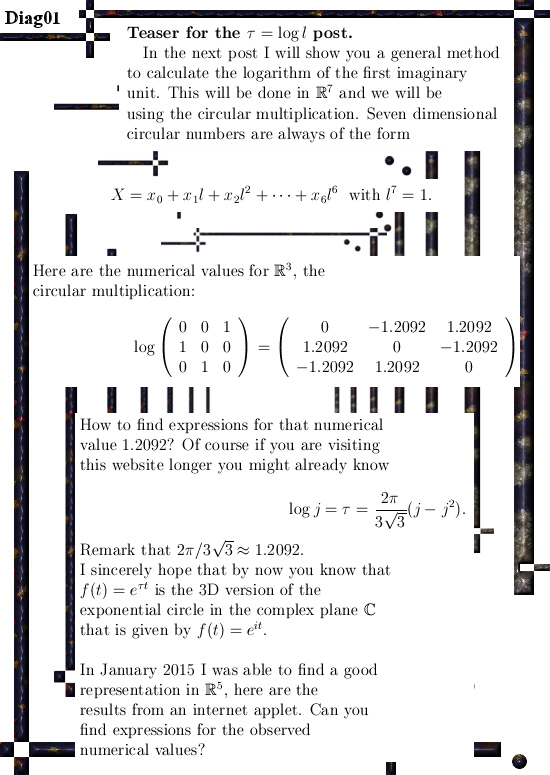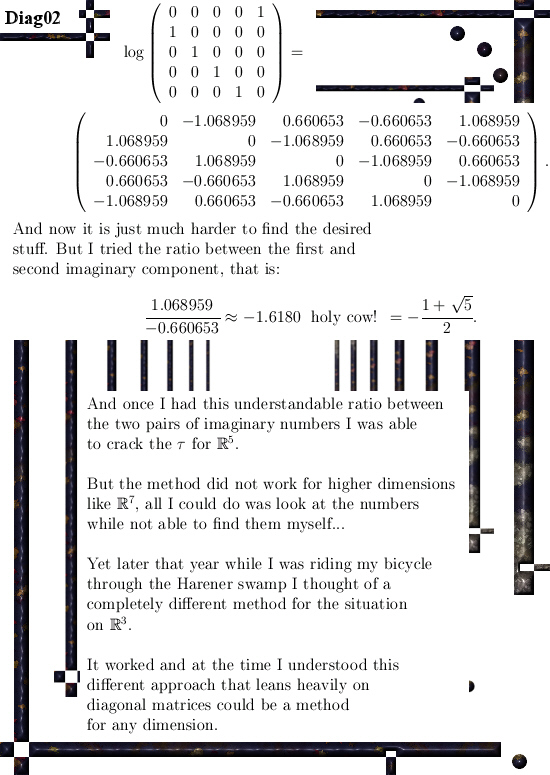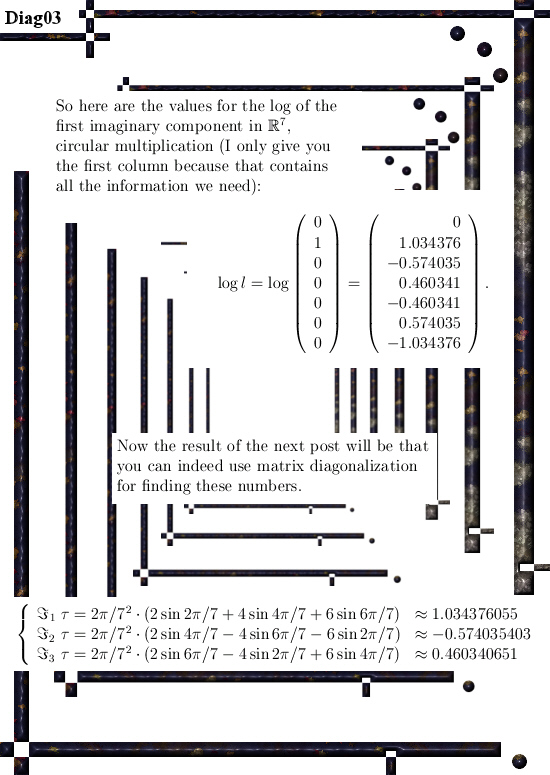So it took some time to find this result, I wasted an entire week using the wrong cut in the complex plane. And that was stupid because I had forgotten my own idea when riding my noble iron horse through the Harener swamps…

The result for the seven dimensional number tau (circular version) as calculated in the next post is a blue print for any dimension although I will never write stuff down like in a general dimension setting because that is so boring to read.

Ok, see you around my dear reader.

# On a way to find more equations so that the 1D existence of exponential curves in all possible dimensions is assured.

In part this post picks up where I left the stuff of the missing equations back in the year 2015. The missing equations are found inside the determinant equation; for this to succeed we must factorize determinant of the matrix representations of higher dimensional numbers. A well known result from linear algebra is that the determinant is also the product of the eigen values; so we need to craft the eigen value functions that for every X in our higher dimensional number space give the eigen values.

These eigenvalue functions are also the discrete Fourier transform of our beloved higher dimensional numbers and these functions come in conjugate pairs. Such a pair form two factors of the determinant and if we multiply them we can get rid of all complex coefficients from the complex plane.

A rather surprising result is the fact that if we subtract a cone equation from a sphere equation we get a cylinder…

This post is also a way of viewing the exponential circles and curves as an intersection of all kinds of geometric objects like the unit sphere, (hyper) cones, (hyper) planes and (hyper cylinders. Usually I represent it all as some analysis but you can take a very geometric approach too.

I have no idea if the shape of the higher dimensional curves is studied as a geometrical object; I suspect this is not the case since the use of complex numbers outside the complex plane is very seldom observed. The professionals just want their tiny fishing bowl (the complex plane) and declare it an Olympic swimming pool…
Well, let it be because these people will never change.

All in all this post is 20 pictures long (size 550 x 775) so it is a relatively long read.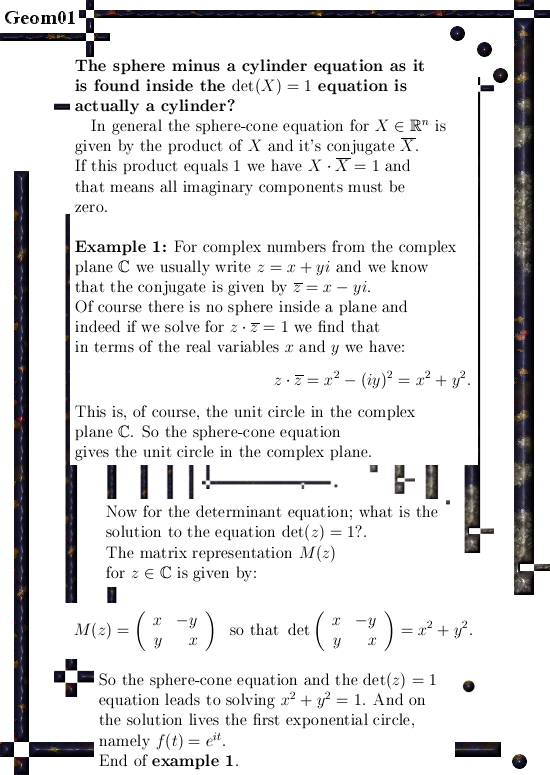The pictures of the graphs were all made with an applet named Animated drawing, here is a link and there you can find it under ´Online calculators and function plotters´±

For example you can cut and paste the next five dimensional equations that represents a hypercone going through all the coordinate axis:

((1/5)*sin(5*x)/sin(x))*((1/5)*sin(5*(x-2*pi/5))/sin(x-2*pi/5)) +
((1/5)*sin(5*(x-2*pi/5))/sin(x-2*pi/5))*((1/5)*sin(5*(x-4*pi/5))/sin(x-4*pi/5)) +
((1/5)*sin(5*(x-4*pi/5))/sin(x-4*pi/5))*((1/5)*sin(5*(x-pi/5))/sin(x-pi/5)) +
((1/5)*sin(5*(x-pi/5))/sin(x-pi/5))*((1/5)*sin(5*(x-3*pi/5))/sin(x-3*pi/5)) +
((1/5)*sin(5*(x-3*pi/5))/sin(x-3*pi/5))*((1/5)*sin(5*x)/sin(x))

The above thing should give identical zero for all x.
An important feature of exponential curves in spaces with an odd number of dimensions is that they all are inside a hyperplane. The hyperplane says the sum of the coordinates is always 1. If you cut and past the next sum of the five coordinate functions you see that you always get one for all x:

((1/5)*sin(5*x)/sin(x)) +
((1/5)*sin(5*(x-pi/5))/sin(x-pi/5)) +
((1/5)*sin(5*(x-2*pi/5))/sin(x-2*pi/5)) +
((1/5)*sin(5*(x-3*pi/5))/sin(x-3*pi/5)) +
((1/5)*sin(5*(x-4*pi/5))/sin(x-4*pi/5))

At last the link to the original update from 2015 where I found the missing equations for the first time. But all I knew they were hidden inside the determinant. A few weeks ago I decided to take a better look and the result is this post.

From 14 July 2015: The missing equations.
http://kinkytshirts.nl/rootdirectory/just_some_math/3d_complex_stuff03.htm#14July2015

# On an old idea that did not work…

Recently I am working on a relatively long post where I try to take a much more geometric approach to finding exponential circles and exponential curves. That post is also going forward from a few years back when I was searching for the so called missing equations.

The problem of the missing equations does not arise until you start working in five dimensions or higher; the equations as generated by the sphere-cone equations are just not enough to end up with a one dimensional curve.
Back in the time I simply took a few weeks until I had found the answer: The missing equations can be found inside the determinant!

For example if you have a 17 by 17 matrix in 17 variables (the so called matrix representations of 17-dimensional number systems), all you have to do is factorize this determinant and from those factors you can craft the extra needed equations.

Weirdly enough you find a hyper plane and a bunch of hyper-cylinders.

So in the next post I try to show you how you can have a very geometric approach to finding the higher dimensional exponential curves as the intersection of a sphere, a hyperplane, a bunch of cones and a bunch of cylinders.

__________

In my old notes I found a mysterious looking line of squares of cosines with their time lags. That was from before I solved the problem and this ‘solution’ has all kinds of faults in it.

That is this small post; it is about something that does not work.
It is just 3 pictures long (550 x 775 pixels):

It was just over two years back I wrote that long update on the other website about the missing equations, I was glad I took those weeks to solve this problem because it is crucial for the development of general higher dimensional theory on this detail.

Here is a link to that old update±

I hope next week I am ready with the new long post, after that I will likely pick up magnetism again because I finally found out what the professionals mean when talking about ´inverted V-s’; that means there is also an electric field accelerating the particles in the aurora’s of earth and Jupiter.

Tiny problem for the professionals: At the Jupiter site, regardless of inverted V-s yes or no, the plasma particles get accelerated anyway…
So that looks like one more victory for Reinko Venema and one more silence from the professional professors.

See ya around!

# How I found the first modified Dirichlet kernel in 5D numbers.

Back in Jan 2014 I was able to solve the circular and complex 5D numbers systems using the so called ‘tau-calculus’. Basically tau-calculus is very easy to understand:

1. Find a basis vector (from an imaginary number) that has determinant +1.
2. Craft analytical expressions that you cannot solve but use internet applets for numerical answers. Or, equivalent:
3. Use an applet to calculate the logarithm of your basis vector once you have put it on a matrix representation.
4. Start thinking long and hard until you have solved the math analysis…

This is easy to understand but I could only do this in three and five dimensional space, seven or eleven dimensional space? It is now 2.5 years later and I still have no clue whatsoever.

Anyway back in the time it was a great victory to find the exponential curves in 5D space. May be some people with insect like minds think that the Euler identity is the greatest formula ever found but let me tell you:
The more of those exponential circles and curves you find, the more boring the complex plane becomes…

In the months after Jan 2014 I wanted to understand the behavior of the coordinate functions that come along those two exponential curves. But the problem kept elusive until I realized I had one more round of internet applets waiting for me.
I had to feed this internet applet for the log of a matrix over 50 matrices and write down the answers it gave me; that was a lot of work because every matrix had 25 entries.

Originally I planned for ‘digesting’ 10 matrices a day so it would be a five day project, but when I finally started somewhere in June 2014 after two days I was ready to sketch my strongly desired coordinate function of the very first coordinate in 5D space.

I still remember sitting at my table and do the drawing and when finished it was so fast that I realized the next: This is a Dirichlet kernel.

And the way I used it, it was so more simple to write down this kernel and if you think how those exponential curves in higher dimensional spaces work with their starting coordinate function and all the time lags that follow… This finding will forever be in the top ten of most perfect math found by your writer Reinko Venema…

__________

Ok, what is in this new post?
Nine pictures of size 550 x 775 pixels containing:
Basic definitions upon 5D complex and circular number &
Tau calculus for the exponential curve &
Explaining how I found the graph of the first modified Dirichlet kernel &
A small quantum physics example related to probability amplitudes &
Some cute integrals that are easy to crack now.

Hope you can learn a bit from it, do not worry if you do not understand all details because even compared to the 2D complex plane or the 3D complex numbers this website is about:
The 5D realm is a space on it’s own!

Have fun reading & thinking upon it: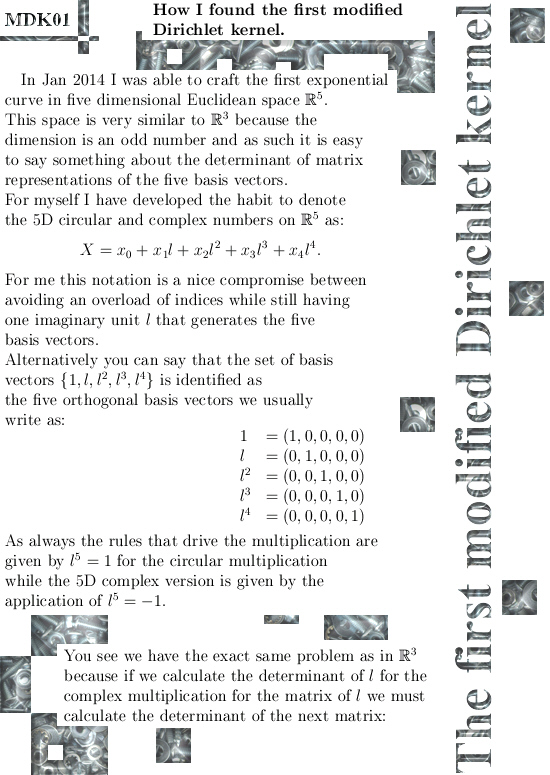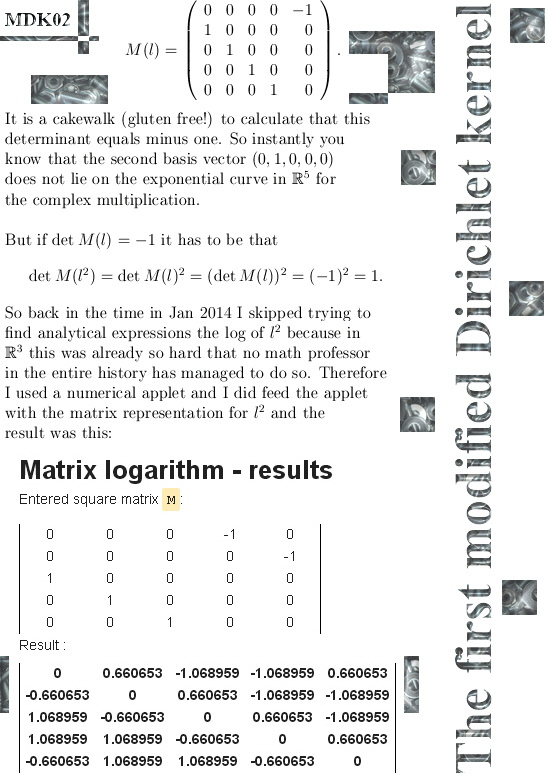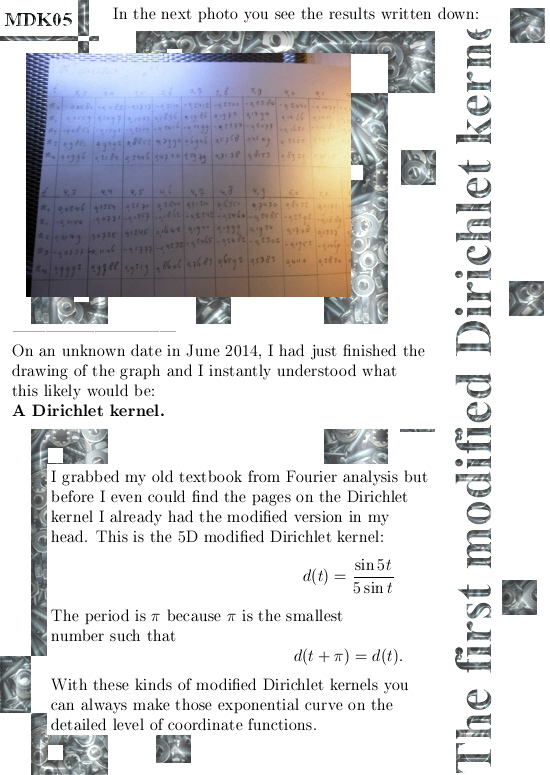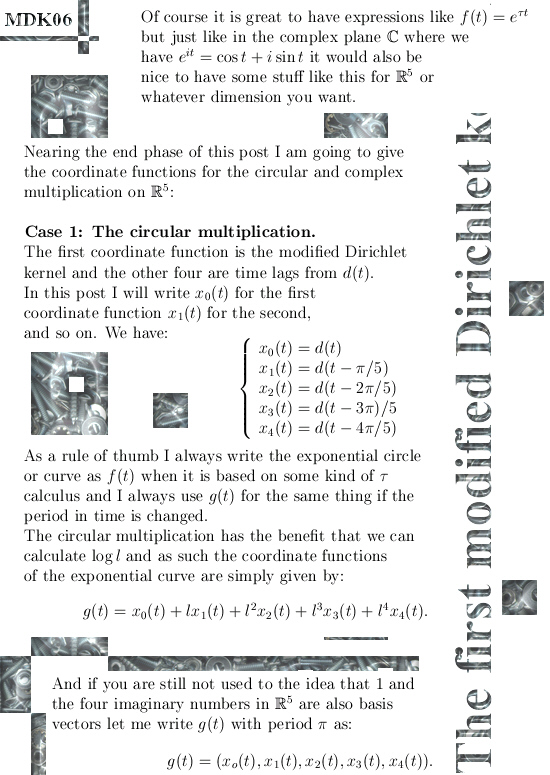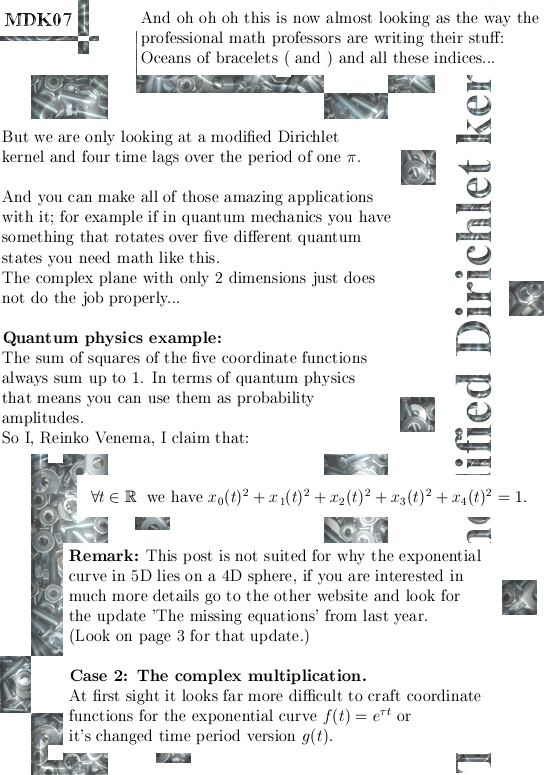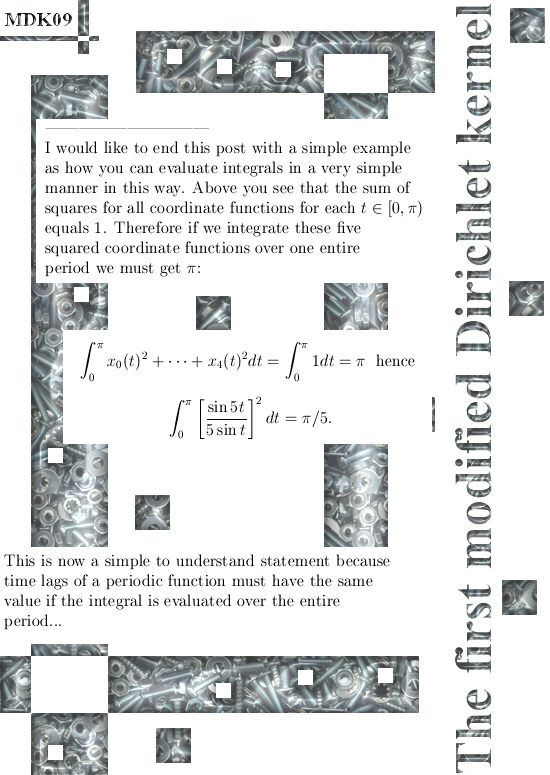A few links of interest are the next:

You need a good applet for the log of a matrix representation if you want, for example, crack the open problem in tau calculus for the 7D complex numbers:
http://calculator.vhex.net/calculator/linear-algebra/matrix-logarithm

My original update on the other website about the 5D number systems from Jan 2014:
http://kinkytshirts.nl/rootdirectory/just_some_math/3d_complex_stuff02.htm#30Jan2014

If you are more interested in those kind of weird looking integrals suddenly easy to solve if you use a proper combination of geometry and analysis, the update from July 2015 upon the missing equations is also worth a visit:
The missing equations.
http://kinkytshirts.nl/rootdirectory/just_some_math/3d_complex_stuff03.htm#14July2015

__________

This is what I more or less had to say, have a nice life or try to get one.
See you in the next post.

# Modified Dirichlet kernels for low dimensions.

What the hydrogen bomb is in the average nuclear arsenal, that is what modified Dirichlet kernels are for higher dimensional complex & circular number systems.
Via the so called tau calculus I was able to achieve results in 3 and 5 dimensional number systems and I really had no hope in making more progress in that way because it gets so extremely hardcore that all hope was lost.

Yet about two years ago I discovered a very neat, clean and very beautiful formula that is strongly related to the Dirichlet kernel known from Fourier analysis. The formula I found was a dressed down version of the original Dirichlet kernel therefore I named it ‘modified Dirichlet kernel’.

This modified kernel is your basic coordinate function, depending on the dimension of the space you are working in you make some time lags and voila: There is your parametrization of your higher dimensional exponential (periodic) curve (only in 2D and 3D space it is a circle).

For myself speaking: this result of finding the modified Dirichlet kernel is for sure in my own top 10 list of most important results found. Not often do I mention other mathematicians, but I would like to mention the name of Floris Takens and without knowing how Floris thought about taking a sample of a time series and after that craft time lags on that, rather likely I would not have found this suburb and very beautiful math…

__________

I haven’t decided what the next post will be about.
It could be stuff like:

• How I found the first modified Dirichlet kernel, or
• Wirtinger derivatives for 3D number systems, or
• Wow man, can you factorize the Laplacian operator form Quantum Mechanics???

But factoring the Laplacian requires understanding 3D Wirtinger derivatives so likely I will show you how I found the very first modified Dirichlet kernel.

__________

This update contains six jpg pictures each about 550 x 775 pixels and two old fashioned animated gif pictures. I tried to keep the math as simple as possible and by doing that I learned some nice lessons myself… Here we go: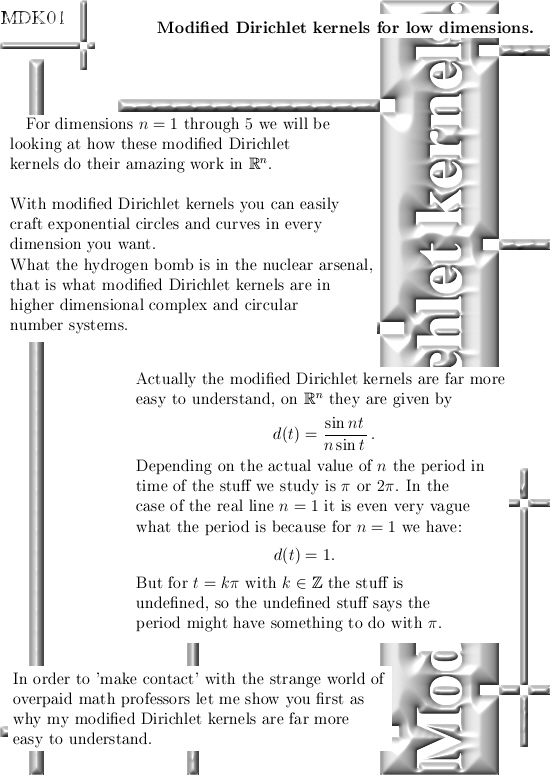This is the animated gif using z = 0 in 3D while this picture is showing the Euler exponential circle: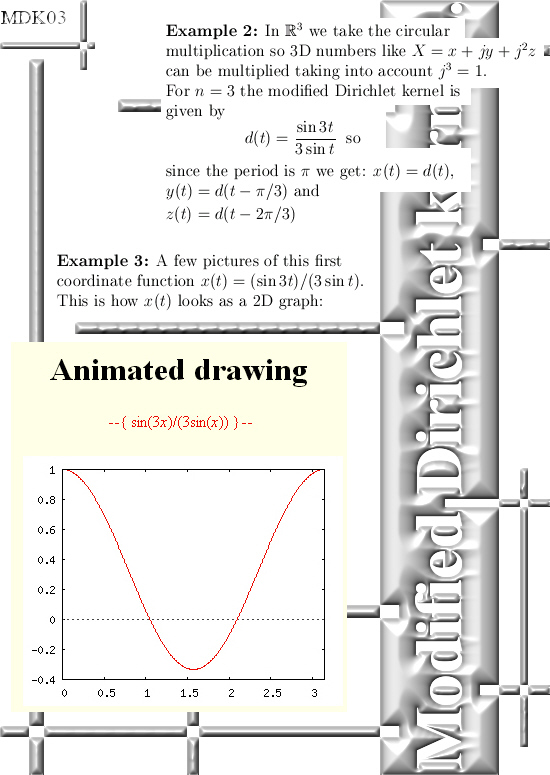Here is an animated gif of how this coordinate function looks when you combine it with the two time lags for the y(t) and z(t) coordinates. Does it surprise you that you get a flat circle?
If it does not surprise you, you do not understand how much math is missing in our human world…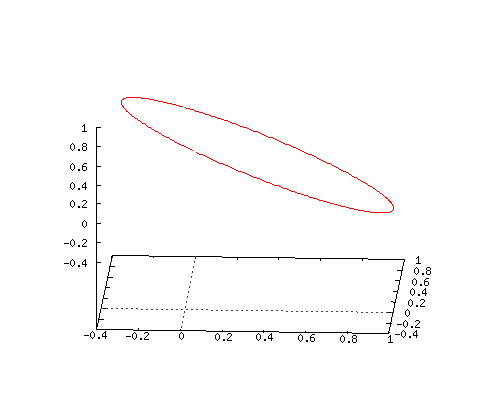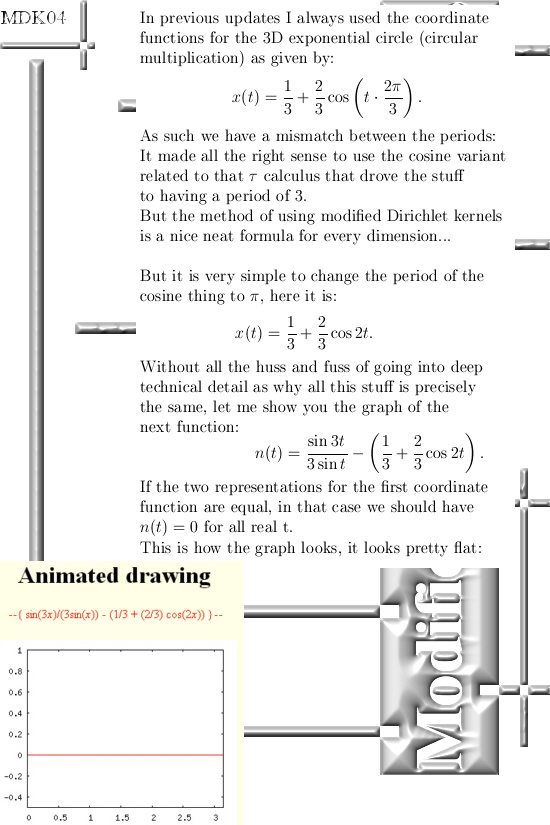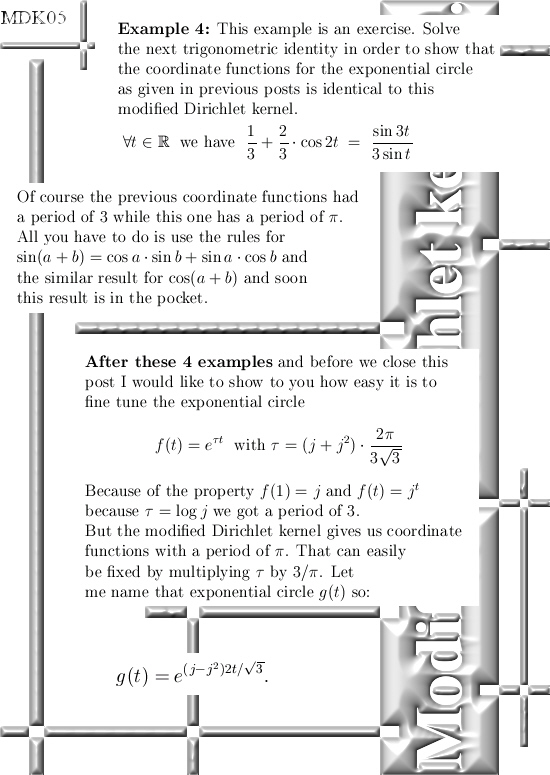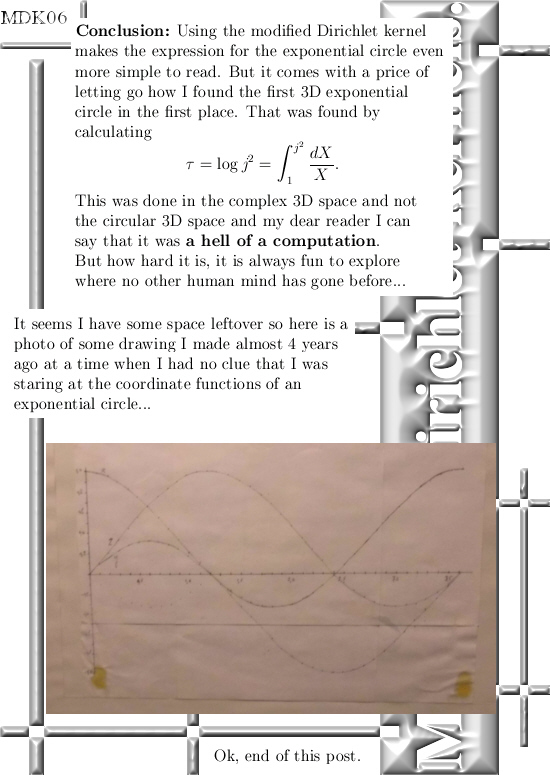In case you are interested in the ‘time lag’ idea as Floris used it, here is a nice Youtube video that gives a perfect explanation. If you apply this time lag idea for example is a 17 dimensional real vector space you get a 17D exponential curve with all of it’s magnificent properties…

Yes, end of this post. See ya around & have a nice life or try to get one.

# An important correction on a very very stupid typo…

Let’s not do difficult: there are typo’s and there are typo’s.

The one kind is for every body directly to understand ‘this is a typo’ and the brain pops up what should be the correct thing standing there.

And there is that kind of typo that is a disaster in people trying to understand what this stuff actually means.

I am sorry I messed things up, but it is like picture six on the previous update on the length of complex numbers that must have been a root cause of much disunderstanding.

In the next picture I show you the correction: in that matrix it now reads log r instead of only r.

Why I was that stupid a few weeks ago is non of your business, but by now stuff is repaired and we no longer have this horrible mistake around any longer…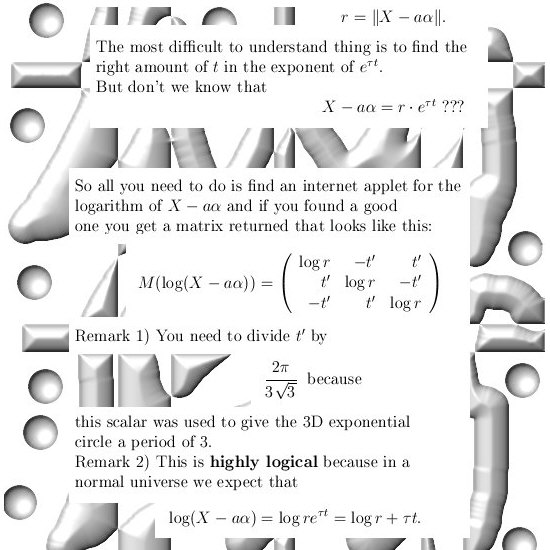Ok, let’s leave it with that. Till updates.

# When did I find the first exponential circle in 3D space?

It was in the Spring of 2013 when I was walking in a nearby park when it suddenly dawned on me that this exponential process that ran through the basis vectors (1, 0, 0), (0, 1, 0) and the z-axis unit vector (0, 0, 1) was periodic.
It could not be anything else because I was capable of calculating the logarithm of the first imaginary unit j.

I remember at first I just did not have a clue it would be a circle, I even had vague fantasies like may be it is a vibrating string where all those string physics professors talk about.

Now this evening I was just Googleing around a little bit when I came across this picture again: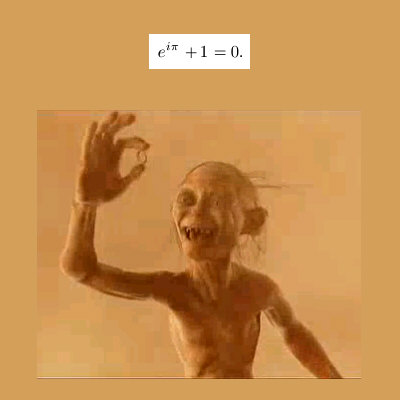It dates back to 30 May 2013 and I used this picture as a joke about how professional math professors look in my fantasy world. Within a week I found that the 3D complex periodic curve was in fact a circle.
So I had to laugh hard about my own joke once more because if I had known the 3D periodic thing would also be a circle I would have made the joke very different… Because one way or the other this picture now also represented me.

__________

You know this last week I am a bit puzzled by what the next post should be, in December 2013 I conducted a good investigation into the roots of unity related to the two exponential circles and because every body knows roots of unity it would a nice started for this website.

On the other website you can find it at the 05 Jan 2014 entry:

http://kinkytshirts.nl/rootdirectory/just_some_math/3d_complex_stuff02.htm#05Jan2013

At the time I was amazed with all the things you can do with the eigen values of the imaginary components j and j squared. From diagonalization to the roots of unity, my theory got definitely air born.

Later in January 2014 I found a new Cauchy integral formula (actually two just like I found two sets of roots of unity each for the admissable forms of 3D multiplication). Also in Jan 2014 I cracked the problem of 5D complex numbers.

By all standards, as far as I can see it; the two months Dec & Jan in that time were the most productive ever.

__________

Now, almost 3 years later finally stuff on Google take off, for example if this day I start searching for the phrase 3dcomplexnumbers it returns back three results from this new website.
Just look at the next screen shot picture: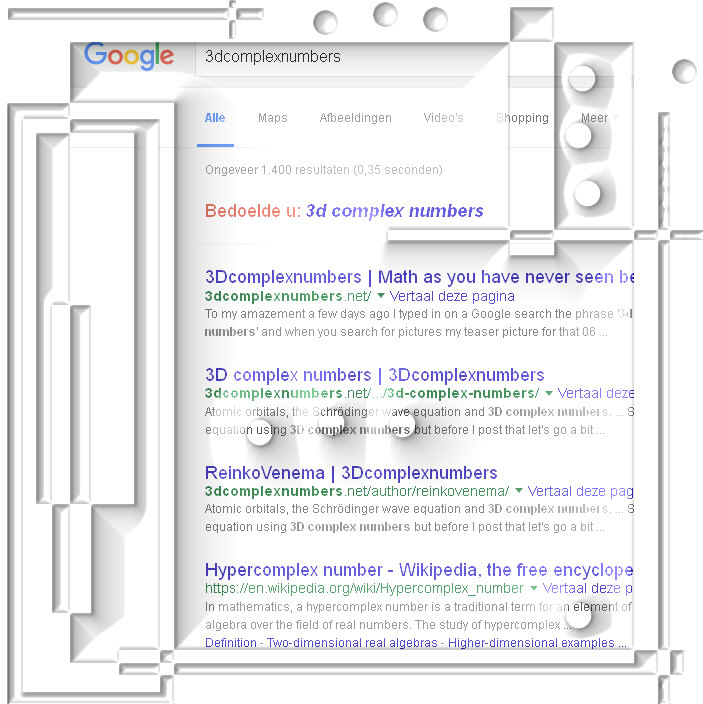So after waiting all these years, finally I begins to look as if stuff starts getting air born on some  bigger scale than before.

Ok, end of this update. As usual till updates!